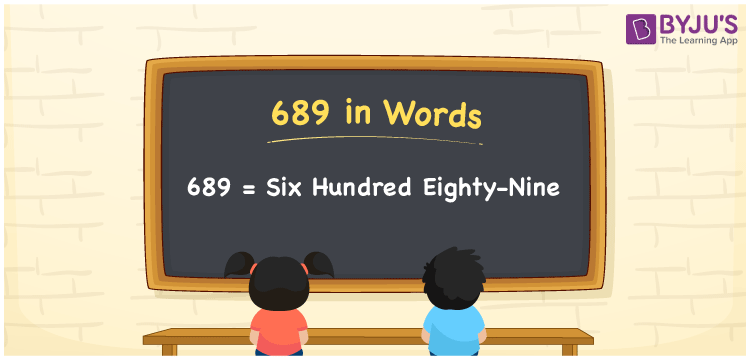# 689 in Words

689 in words is written as Six hundred eighty-nine. In both the International System of Numerals and the Indian System of Numerals, 689 is written as Six hundred eighty-nine. The number 689 is a Cardinal Number as it represents some quantity. For example, “She spent 689 rupees”.

 689 in Words Six hundred eighty-nine Six hundred eighty-nine in Number 689

## 689 in English Words

We write 689 in English Words using the letters of the English alphabet. Therefore, we read 689 in English as “Six hundred eighty-nine.”## How to Write 689 in Words?

To write 689 in words, we shall use the place value chart. In the place value chart, write 6 in the hundreds, 8 in the tens, and 9 in the ones, respectively. Now let us make a place value chart to write the number 689 in words.

 Hundreds Tens Ones 6 8 9

Thus, we can write the expanded form as

6 × Hundred + 8 × Ten + 9 × One

= 6 × 100 + 8 × 10 + 9 × 1

= 600 + 80 + 9

= 689

= Six hundred eighty-nine.

689 is a natural number, the successor of 688 and the predecessor of 690.

689 in words – Six hundred eighty-nine

• Is 689 an odd number? – Yes
• Is 689 an even number? – No
• Is 689 a perfect square number? – No
• Is 689 a perfect cube number? – No
• Is 689 a prime number? – No
• Is 689 a composite number? – Yes

## Frequently Asked Questions on 689 in Words

Q1

### How to write 689 in words?

689 in words is written as Six hundred eighty-nine.
Q2

### How to write 689 in the International and Indian System of Numerals?

In both, the system of numerals, 689 in words, is written as Six hundred eighty-nine.
Q3

### What is the preceding number of 689?

The number that precedes 689 is 688.